《PowerBI火力全开+可视化+DAX神功》完整视频教程

https://www.ixigua.com/6976409163886756383

YTD：当年累计，Year To Date的简写

QTD：当季度累计，Quarter To Date的简写

MTD：当月累计，Month To Date的简写

【1】语法：年初至今

DATESYTD(日期,<上一年的截止日期>)

`【新建表】年初至今 = DATESYTD('Sheet1'[日期],"6-30")【新建表】年初至今 = DATESYTD('Sheet1'[日期],"2021/6/30")【新建表】年初至今 = DATESYTD('Sheet1'[日期],"2021-6-30")【新建表】年初至今 = DATESYTD('Sheet1'[日期],"2021年6月30日")`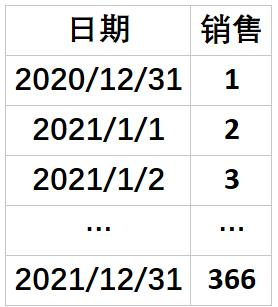`【新建表】年初至今 = DATESYTD('Sheet1'[日期],"2020/12/31")【新建表】年初至今 = DATESYTD('Sheet1'[日期])`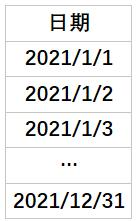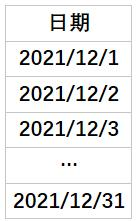`【新建表】年初至今 = DATESYTD('Sheet1'[日期],"2021/12/31")【新建表】年初至今 = DATESYTD('Sheet1'[日期],"2021-12-31")【新建表】年初至今 = DATESYTD('Sheet1'[日期],"2021年12月31日")`

`【新建表】年初至今 = DATESYTD('Sheet1'[日期],"12-31")【新建表】年初至今 = DATESYTD('Sheet1'[日期])`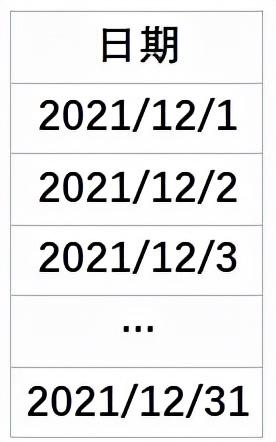【2】语法：月初至今与季初至今 （没有第2个参数）

`【新建表】月累计语法=DATESMTD(<日期>)``【新建表】季度累计语法=DATESQTD(<日期>)`【3】优化上节课案例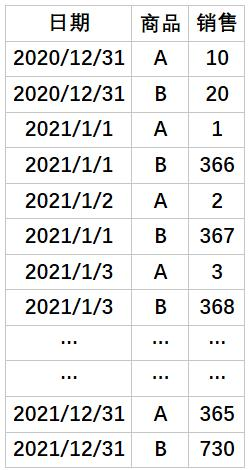`日期表 = ADDCOLUMNS(CALENDAR(FIRSTDATE('Sheet1'[日期]),LASTDATE('Sheet1'[日期])),"年", YEAR ( [Date] ),"季度", ROUNDUP(MONTH([Date])/3,0),"月", MONTH([Date]),"周", weeknum([Date]),"年季度", year([date]) & "Q" & ROUNDUP(MONTH([Date])/3,0),"年月", year([Date]) * 100 + MONTH([Date]),"年周", year([Date]) * 100 + weeknum([Date]),"星期几", WEEKDAY([Date]))`

`总销售 = sum(Sheet1[销售])度量值3 = calculate([总销售],FILTER(all('日期表'),'日期表'[Date]<=max('日期表'[Date]) && '日期表'[年]=2021))年初至今 = calculate([总销售],DATESYTD('日期表'[Date]))`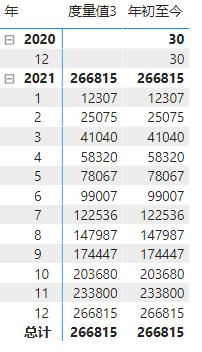`年初至今 = calculate([总销售],DATESYTD('日期表'[Date]),'日期表'[年]=2021)`

Ps: 上节课没有白听吧？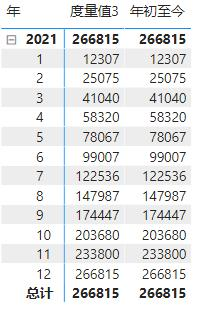《火力全开》笔记27课，向大家说过，这个函数是可以被替代的。

【1】语法：年初至今=TOTALYTD(表达式或度量值,日期, 筛选器,上一年结束日期)

`TOTAL年初至今 = TOTALYTD([总销售],'日期表'[Date],'日期表'[年]=2021)`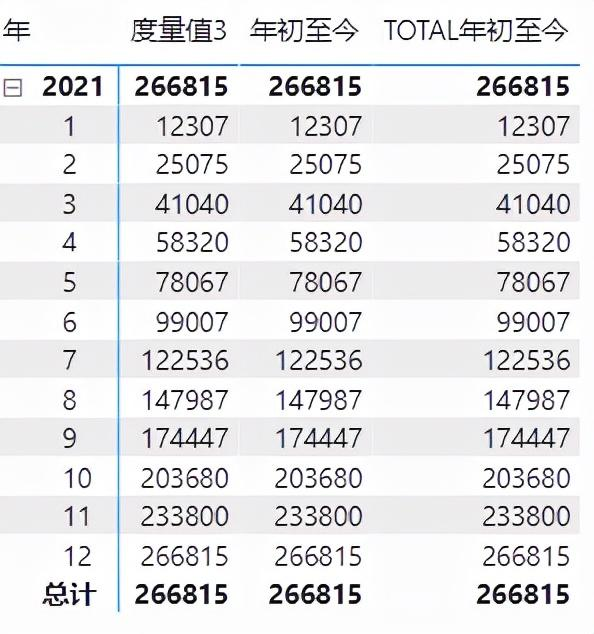【2】语法：月初至今=TOTALMTD(表达式或度量值,日期, 筛选器)

【3】语法：季初至今=TOTALQTD(表达式或度量值,日期, 筛选器)

`【度量值】TOTAL月初至今1 = TOTALMTD([总销售],'日期表'[Date],'日期表'[年]=2021)【度量值】TOTAL月初至今2 = CALCULATE([总销售],DATESMTD('日期表'[Date]),'日期表'[年]=2021)`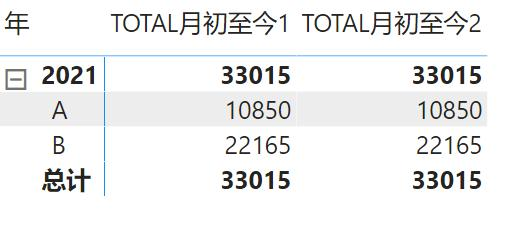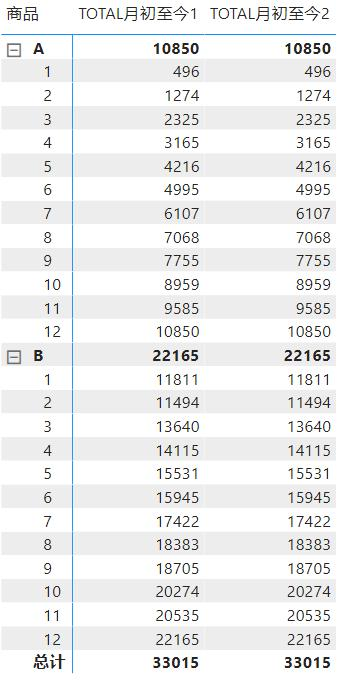`TOTAL月初至今1 = TOTALMTD([总销售],'日期表'[Date],'日期表'[年]=2021 && '日期表'[月]=12)TOTAL月初至今2 = CALCULATE([总销售],DATESMTD('日期表'[Date]),'日期表'[年]=2021,'日期表'[月]=12)`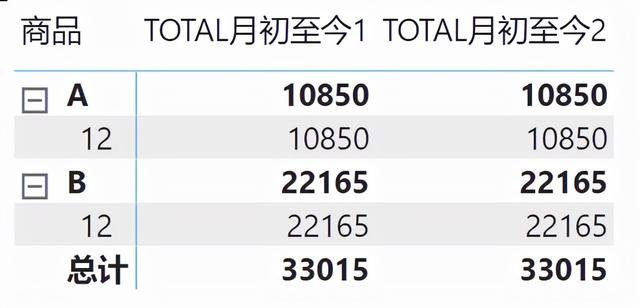PS: 使用切片器也可以